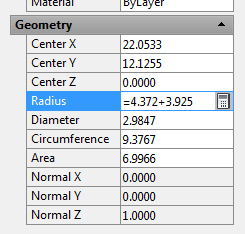AutoCAD 2015 and AutoCAD LT 2015 Bible

The most comprehensive AutoCAD book around!# Let AutoCAD do the math

There are several ways you can let AutoCAD do some quick arithmetic for you when you need a distance. Let’s say you’re drawing a line and you want to use direct distance entry to specify the length. What you know is that that line should be 4.372 plus 3.925. Of course, you could use a hand calculator or (gasp!) take out a pencil and paper and do the math.

## Use an AutoLISP expression

Tony Dakin suggested the following method, which uses an AutoLISP expression. At the Specify next point or [Undo]: prompt, enter the following:

(+ 4.372 3.925)

2. Next comes the operator, such as. + – * or /.
3. Add a space
4. Add the first number
5. Another space
6. Add the second number
7. Close the parentheses.

## Use the CAL command

There’s another way: you can use the old CAL command. Here’s how:

At the Specify next point or [Undo]: prompt, enter ‘cal and press Enter.

At the >>>> Expression: prompt, simply enter 4.372 + 3.925 and press Enter.

Enter math expressions in dialog box text boxes and the Properties palette

Did you know that you can enter expressions in dialog boxes and the Properties palette? Just type the expression, such as 4.372+3.925 and press Alt-Enter (don’t forget to press the Alt key!) You may have to press Enter to complete the change. For example, you can use this method to change the radius of a circle in the Properties palette.For this to work, the CALCINPUT system variable must be set at 1, which is the default setting.

Related tips:

### 2 comments to Let AutoCAD do the math

•Ralph Tulis

Okay, I get this. Been using NN00/254 to enter centimeters as inches for years, where NN is the distance in cm. As long as the numerator and denominator are integers, this works practically everywhere.

I get using ‘cal as well – if all you want is the value. But how can I use an expression when trying to enter line line segment by bearing? I very often work with drawings using decimal feet. I want to convert to inches as I go by simply multiplying by 12. But how can this be done when trying to draw a line segment by distance@bearing, i.e. 144<S45D0'0"E?

•Mark

Switch to Bentley Microstation. They’ve been able to do this from any key-in field for about 20 years.